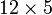# Direct product of A4 and Z5

View a complete list of particular groups (this is a very huge list!)[SHOW MORE]

## Definition

This group is defined as the external direct product of alternating group:A4 and cyclic group:Z5.

## Arithmetic functions

Want to compare and contrast arithmetic function values with other groups of the same order? Check out groups of order 60#Arithmetic functions
Function Value Similar groups Explanation
order (number of elements, equivalently, cardinality or size of underlying set) 60 groups with same order order of direct product is product of orders: the order is thus$12 \times 5$, where$12 = 4!/2$ is the order of alternating group:A4 and$5$ is the order of cyclic group:Z5.
exponent of a group 30 groups with same order and exponent of a group | groups with same exponent of a group exponent of direct product is lcm of exponents: the exponent is$\operatorname{lcm}\{ 6,5 \}$. Also, note that for any non-cyclic group of order 60, the exponent must be 30, because exponent of a finite group has precisely the same prime factors as order.
minimum size of generating set 2 groups with same order and minimum size of generating set | groups with same minimum size of generating set minimum size of generating set of direct product of groups of coprime order equals maximum of minimum size of generating set of each factor: in this case, we get$\max \{ 2, 1 \} = 2$.
derived length 2 groups with same order and derived length | groups with same derived length derived length of direct product is maximum of derived lengths: we get$\max \{ 2,1 \} = 2$.

## GAP implementation

### Group ID

This finite group has order 60 and has ID 9 among the groups of order 60 in GAP's SmallGroup library. For context, there are 13 groups of order 60. It can thus be defined using GAP's SmallGroup function as:

SmallGroup(60,9)

For instance, we can use the following assignment in GAP to create the group and name it$G$:

gap> G := SmallGroup(60,9);

Conversely, to check whether a given group$G$ is in fact the group we want, we can use GAP's IdGroup function:

IdGroup(G) = [60,9]

or just do:

IdGroup(G)

to have GAP output the group ID, that we can then compare to what we want.

### Other descriptions

Description Functions used
DirectProduct(AlternatingGroup(4),CyclicGroup(5)) AlternatingGroup, CyclicGroup